# Identification and utilization of arbitrary correlations in models of recombination signal sequences

## Abstract

### Background

A significant challenge in bioinformatics is to develop methods for detecting and modeling patterns in variable DNA sequence sites, such as protein-binding sites in regulatory DNA. Current approaches sometimes perform poorly when positions in the site do not independently affect protein binding. We developed a statistical technique for modeling the correlation structure in variable DNA sequence sites. The method places no restrictions on the number of correlated positions or on their spatial relationship within the site. No prior empirical evidence for the correlation structure is necessary.

### Results

We applied our method to the recombination signal sequences (RSS) that direct assembly of B-cell and T-cell antigen-receptor genes via V(D)J recombination. The technique is based on model selection by cross-validation and produces models that allow computation of an information score for any signal-length sequence. We also modeled RSS using order zero and order one Markov chains. The scores from all models are highly correlated with measured recombination efficiencies, but the models arising from our technique are better than the Markov models at discriminating RSS from non-RSS.

### Conclusions

Our model-development procedure produces models that estimate well the recombinogenic potential of RSS and are better at RSS recognition than the order zero and order one Markov models. Our models are, therefore, valuable for studying the regulation of both physiologic and aberrant V(D)J recombination. The approach could be equally powerful for the study of promoter and enhancer elements, splice sites, and other DNA regulatory sites that are highly variable at the level of individual nucleotide positions.

## Background

### Modeling variable DNA sequence sites

The set of binding sites for a single DNA-binding protein can be highly variable [1,2], and the degree of nucleotide variation tolerated generally differs from position to position within a site [3,4,5]. This sequence diversity can have important functional consequences as the affinity between regulatory proteins and their binding sites is modulated by changes in binding-site sequence . Large datasets of related DNA sites are now available [3,4,6,7]. Currently, more than 100 prokaryotic and eukaryotic genomes have been completely sequenced, permitting cross-species comparisons of even larger sequence assemblies than collected here (see, for example [8,9]). Computational approaches can therefore be used to detect and model the sequence patterns present within the binding-site variability. The most useful models of variable DNA sites provide classification algorithms for identifying functional sites as well as insights that can help elucidate the relationship between the structure and function of these sites.

Variable DNA sequence sites are frequently described by a consensus sequence  or a weight matrix [11,12,13]. Consensus sequences have limited utility for even moderately variable sites because they preserve little or no information about the variability within the sequences they characterize. Differences from consensus are quantified by counting the number of mismatched positions, making no distinction between mismatches that abolish function of the site and those that modulate function.

Weight matrices were introduced to characterize transcription and translation initiation sites in Escherichia coli  and have become standard for characterizing binding sites for transcription factors. A weight matrix is a two-dimensional matrix in which each row corresponds to one of the four nucleotides and each column corresponds to one position in the site being described . The elements of the matrix are the observed counts for each nucleotide at each position in an alignment of the DNA sites , or some function of these counts (reviewed in [13,14]). Any sequence can be scored by summing the matrix elements corresponding to the sequence , thereby quantitatively rating the sequence's functional potential.

Weight matrices can be used to scan genomic DNA and determine statistically significant matches to the sequence pattern described by the matrix . Putative sites are scored by the weight matrix, so the number of false positives and false negatives can be balanced by choosing an appropriate threshold score defining functional sites. The scores of functional sites are sometimes correlated with their level of function [11,14,15,16,17,18,19,20,21,22,23].

Weight matrices are typically based on order zero Markov chains, meaning they assume that individual base pairs in the binding site affect binding affinity independently. Weight-matrix models based on order one Markov chains assume that adjacent positions are correlated [15,24,25,26,27]. Ponomarenko et al.  constructed weight matrices for many different transcription factor binding sites using mono-, di-, and trinucleotide motifs allowing correlation between non-adjacent positions by specifying X1NX3 and X1NX3NX5 motifs, where Xi is the nucleotide at position i of the motif and N is any nucleotide. While this approach allows for correlation between more than two non-adjacent positions, the motifs may still be unnecessarily restrictive. The apposition of distant binding-site positions can be important to recruitment of the binding protein, for example, through formation of DNA secondary structure [29,30,31,32], or to the interaction of the binding site with its binding protein . Therefore correlation between distant positions is expected.

Burge and Karlin  used hypothesis testing via χ2 tests to detect significant correlations between any two positions and introduced a model-building procedure, maximal dependence decomposition, to account for the dependencies. For each significant correlation, this method partitions the dataset into subsets of sequences exhibiting no correlation; the final model is a composite of weight matrices (order zero), one for each subset. Our model-building technique is based on cross-validation criteria rather than on hypothesis testing per se, and we use a more general model class for DNA sequences.

Models that allow for pairwise correlations between positions were developed in the context of Bayes networks by Cai et al. . Bayes networks represent a large class of models, and in fact, our model can be recast as a Bayes network, although it is not now formulated in those terms.

We have developed an approach to determine the correlation structure present in a set of variable sequence sites. For each position in the site, we identify all other correlated positions placing no restrictions on the number or spatial relationship of correlated positions in the site. Our approach determines, from all possible combinations of disjoint probability distributions, the set of distributions that most effectively distinguishes functional sites from non-functional sequences. Although the family of models we consider is very large, we proceed by model selection rather than hypothesis testing. The selected probability function is the product of these disjoint probability distributions. The natural logarithm of this probability function can be used as a score for any sequence, thereby recognizing the presence of evolutionarily conserved nucleotide associations. We have applied this approach to recombination signal sequences (RSS), a set of DNA binding sites necessary for specific immunity.

### Recombination signal sequences

Specific immunity depends on the ability of B and T lymphocytes to recognize antigens, molecular identifiers of pathogens . The immune system does not anticipate which antigen will be encountered, but remarkable genetic mechanisms have evolved that generate diverse antigen-receptor repertoires. The primary generative mechanism is V(D)J recombination, the rearrangement of B-cell receptor (BCR) or T-cell receptor (TCR) V, D, and J gene segments to form functional genes; the resulting combinatorial and junctional diversity can produce around 1014 BCR specificities and around 1018 TCR specificities in one animal . This extraordinary plasticity has a price: V(D)J recombination can produce self-reactive receptors leading to autoimmune disease [38,39] and may err to create oncogenic chromosomal translocations .

V(D)J recombination proceeds by the introduction of double-strand DNA breaks (reviewed in ). To prevent DNA breaks that would cause cellular damage, the V(D)J recombinase must be specifically targeted to the Bcr and Tcr loci. This targeting is regulated primarily by binding of RAG1 , an enzyme in the recombinase complex, to the RSS adjacent to each V, D and J gene segment . RSS were initially identified as a conserved heptamer (consensus CACAGTG) and a conserved nonamer (consensus ACAAAAACC) separated by a less conserved spacer of either 12 ± 1 or 23 ± 1 base-pairs (bp) . The initial CAC of the heptamer is highly conserved, but all remaining positions show moderate to very low levels of conservation (reviewed in ).

RSS variability has important functional consequences: the efficiency with which each RSS mediates recombination depends on its sequence . There is strong evidence that differing recombination efficiencies of RSS result in the biased utilization of their associated gene segments (reviewed in [47,48]). In addition, the biases in gene segment use observed in the post-selection TCR repertoire are consistent with the biases observed before selection, suggesting that the primary immune repertoire may be genetically patterned .

The high level of diversity among RSS makes it very difficult to evaluate their recombinogenic potential. RSS not associated with a V, D or J gene segment but participating in aberrant (illegitimate) V(D)J recombination are particularly difficult to recognize. These non-physiologic RSS are important because of their potential involvement in oncogenic chromosomal translocation and receptor editing, a process that alters the specificity of autoreactive receptors. Non-physiologic RSS that have simply arisen by chance can be referred to as fortuitous RSS whereas those thought to have descended from the same ancient transposon as physiologic RSS can be referred to as cryptic (cRSS) [49,50]. Generally, the origin of a non-physiologic RSS is not known; for simplicity, we will refer to both types as cryptic.

To understand the role of RSS variability in the formation of the primary immune repertoire and of cRSS in illegitimate V(D)J recombination, it is necessary to quantify the genetic variability among physiologic RSS and the relationship between this variability and the regulation of recombination. Allowing for each of the 4 nucleotides at just 10 of the 28 or 39 RSS positions results in over 106 different signals - it is not feasible to measure the efficiency of all potential RSS experimentally. Statistical models of RSS variability that allow prediction of recombination efficiency are essential to furthering our understanding of the biological function of RSS variability.

One estimate of cRSS frequency in mammalian genomes is one in every 600 bp . Promiscuous recombination at this frequency, however, would result in significant damage to the cell. This suggests that complex patterns underlie the high level of nucleotide diversity observed at individual positions, thereby increasing the specificity of the signal governing recruitment of the V(D)J recombinase.

We find significant correlations between nucleotide positions in RSS, suggesting they act cooperatively and that nucleotide associations are conserved. We used our model selection procedure to determine the best models of RSS correlation structure, one model for 12-bp spacer RSS (12-RSS) and one for 23-RSS. We also modeled both types of RSS with order zero and order one Markov chains. The scores from all six models are highly correlated with measured recombination efficiencies, but the models arising from our technique are much better than the Markov models at identifying physiologic and cryptic RSS in genomic DNA.

## Results

### Mouse RSS show limited sequence conservation

We analyzed 356 physiologic mouse RSS (see Methods). While 62% (219/356) of these RSS contain a consensus heptamer, just 16% (57/356) contain a consensus nonamer and only 13% (48/356) contain both a consensus heptamer and a consensus nonamer. To measure the level of nucleotide diversity at individual RSS positions, we computed the entropy (H i )  for each position i in an alignment of the 201 12-RSS and an alignment of the 155 23-RSS (Figure 1a). A strictly conserved position in which no variation is tolerated has H i = 0, while a position in which the four nucleotides are observed at nearly uniform frequencies has H i = 1.

The signal specified by the individual RSS positions is very low, especially in 23-RSS (Figure 1a). The average entropy is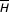12 = 0.50 for 12-RSS and23 = 0.67 for 23-RSS; for a randomly generate string of A, G, C, and T characters, the expected value foris one. The patterns of nucleotide diversity at individual positions are similar for both 12- and 23-RSS: the initial CAC of the heptamer is invariant, the remaining four positions of the heptamer are moderately diverse, the spacer is highly diverse, and the A tract of the nonamer (positions 5 through 7) is better conserved than the other nonamer positions (Figure 1a). The average entropy for 23-RSS is higher than for 12-RSS, primarily because the 23-RSS nonamers are much more diverse than 12-RSS nonamers (N23 = 0.59;N12 = 0.35; Figure 1a).

It is known from experimental studies that nucleotide substitutions at highly diverse positions influence recombination efficiency [46,52]. That a given RSS position can be both highly variable and exhibit strong effects on signal efficiency appears contradictory. The contradiction can be reconciled, however, by hypothesizing correlations between nucleotides in RSS: a nucleotide substitution at one position may be compensated for by a change at another position in the RSS.

### Significant pairwise correlations exist between positions in the RSS

To test for correlation between pairs of positions in the RSS, we computed the mutual information, MIi,i,  between all pairs of positions i and i' in an alignment of the 201 physiologic mouse 12-RSS and in an alignment of the 155 physiologic mouse 23-RSS (see Methods) (Figure 1b). Statistically significant MIi,i' values result when there is a correlation between nucleotide frequencies at the two positions, indicating selection pressure to maintain (or avoid) a particular dinucleotide.

In 12- and 23-RSS, we detect statistically significant correlations between positions in those regions of the RSS exhibiting high levels of position-wise entropy. These are the spacer, especially the nonamer-proximal half, and the positions of the heptamer and nonamer that are adjacent to the spacer (Figure 1b). In general, correlation between two positions decreases as a function of distance, but there are examples of strong correlation between widely separated positions (Figure 1b). For example, we observe 12-RSS with A at positions 8 and 19 at a much higher frequency (0.38) than expected (0.11) from the independent frequencies of A at each position. In addition, we find that the levels of correlation are higher in 23-RSS than in 12-RSS (Figure 1b); this is an especially interesting observation given that 23-RSS are much more variable than 12-RSS (Figure 1a).

### Statistical models of 12- and 23-RSS determine the relevant correlation structure

The MI computations give evidence for strong pairwise correlations, including between distant positions, and correlations may exist between any number of positions. We therefore developed statistical models, one for 12-RSS and one for 23-RSS, that can account for correlations between arbitrary positions without regard for their distance from each other or the number of correlated positions. The models were developed using a procedure that selects from all possible combinations of disjoint probability distributions the set of marginal (for one position) and joint (for multiple positions) distributions that most distinguishes physiologic RSS from other nucleotide sequences of the same length. For example, positions 8 and 19 in 12-RSS are highly correlated (Figure 1b). They could be included in the model either through the two marginal probability distributions - the distribution over the four nucleotides at position 8 and the distribution over the four nucleotides at position 19, or through one joint probability distribution - the distribution over the 16 pairs of nucleotides at the pair of positions. Each model computes a score for RSS information content: RIC12 for 28-bp sequences and RIC23 for 39-bp sequences; the formation of joint probability functions is determined by maximizing the mean score for physiologic RSS.

RIC12 = ln [P1P2P3,15,25P4,5P6,28P7,8,19P9,26P10,12P11,27P13,14,23P16,17,18P20,21,22P24]

where P1 is the marginal probability function for position 1 and P3,15,25 is the joint probability function for positions 3,15 and 25. The presence of the joint probability function in the model indicates that these three positions are mutually correlated. The selected 23-RSS model is:

RIC23 = ln [P1P2P3P4,14P5,39P6P7,24,25P8,9,21P10,16P11,12P13,22P15,23P17,18P19,27,30,31,32,33,37P20,26P28,29P34,38P35,36].

Most of the marginal probabilities have been merged to form joint probability functions, but the order in which the joint functions were formed reflects the relative strength of the correlations between the corresponding positions: positions with large MI are grouped early in model selection (data not shown). The groups of positions with the strongest correlations are (7,8,19), (16,17,18), and (20,21,22) in 12-RSS (Table 1) and (19,27,30,31,32,33,37), (8,9,21), and (7,24,25) in 23-RSS (Table 2). For both 12- and 23-RSS, the positions exhibiting the strongest cooperative influence lie in the nonamer-proximal half of the spacer and in the heptamer and nonamer positions adjacent to the spacer (Figure 2). Interestingly, these positions overlap substantially with positions exhibiting ethylation/methylation interference in RSS complexed with RAG1/RAG2 (Figure 2 and ) suggesting that the correlations detected by the models are relevant to RAG-RSS interaction.

### The selected models were compared with order zero and order one Markov models

To determine whether the correlation structure detected by our model selection procedure improves RSS recognition and evaluation, we constructed weight matrix models based on order zero or order one Markov chains. Order zero Markov models (WM012 for 12-RSS and WM023 for 23-RSS) assume that all RSS positions are independent and are therefore computed from marginal probability distributions. The score for an N-bp sequence is computed as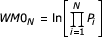where P i is the probability of observing nucleotide X at position i. Order one Markov models (WM112 and WM123) assume that adjacent positions are correlated (the identity of the nucleotide at position i depends on the nucleotide at position i - 1) and are computed from conditional probability distributions: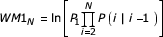where P1 is the marginal probability distribution for position 1, and P(i|i - 1) is the probability of observing nucleotide X at position i given the nucleotide at position i - 1.

For physiologic 12- and 23-RSS, the score distribution is shifted toward higher scores for models that include correlation (RIC and WM1) (Table 3, Figure 3). The difference is most striking for 23-RSS (Table 3, Figure 3). The mean scores for the physiologic 12-RSS are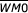12 = -19.84,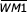12 = -18.49, and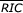12 = -18.47 (Table 3); the mean scores for physiologic 23-RSS are23 = -37.07,23 = -31.51, and23 = -32.39 (Table 3). There is relatively little change in the value of low scores across models, so as the correlation structure of the models increases in complexity, the increase in high scores results in an increase in the score range (Table 3 and Figure 3).

### Functional RSS are best discriminated by RICscores

To test whether functional RSS can be recognized by the sequence properties captured in the models, we characterized the score distributions for non-RSS DNA (Figure 4). These background distributions are characterized by their means and ranges. WM0, WM1 and RIC scores were computed for all 28- and 39-bp segments in a 212,128-bp fragment of mouse chromosome 8 (AC084823) containing no known RSS. We also characterized the background distributions by computing scores for a pseudorandom string (PRS) of A, T, C and G the same length as sequence AC084823 and having the same nucleotide-usage frequencies.

As expected, the WM0 score distributions for AC084823 and the PRS are almost identical for both 28- and 39-bp segments (Table 4, Figure 4). For the models that account for correlation, however, scores from genomic DNA are higher on average than those computed for the PRS (Table 4, Figure 4). This is especially true for the WM1 models, indicating that, whereas both the WM1 and RIC models are influenced by correlations present in physiologic DNA, the correlations detected by the RIC models are more specific to RSS. Importantly, the distributions for RIC scores are always shifted toward lower scores than the corresponding WM0 and WM1 distributions (Table 4, Figure 4).

We next compared across models the frequency of signal-length segments in AC084823 that score above a given threshold. To determine test thresholds for a given model, we ranked the physiologic RSS by score in ascending order and took the lowest 5% of scores under each model as the test thresholds for that model (Table 5, Figure 5). For example, the lowest RIC12 is -48.16. It has rank zero, and zero physiologic RSS have a lower RIC12. Of 212,101 (0.00414) 28-bp segments in AC084823, 859 have RIC12 > -48.16. Similarly, threshold zero under the WM112 model is -46.32, and 2,372 of 212,101 (0.01118) 28-bp segments in AC084823 have WM112 > -46.32. Under each model, the frequency of physiologic RSS scoring below threshold estimates the probability of failing to recognize a functional RSS and is a measure of the model's sensitivity. The frequency of non-RSS scoring above threshold estimates the probability of classifying non-RSS as functional and is a measure of the model's specificity. Both numbers should be small. The high-scoring non-RSS segments may support recombination, however, and, if so, would be classified as cRSS. Those not supporting recombination would be counted as false positives.

In general we find that the WM0 models predict the highest number of RSS in AC084823, and the RIC models predict the fewest (Table 5, Figure 5). Of 20 test thresholds, there are three for which RIC does not predict the smallest number of RSS: for the 12-RSS models, the score for the ninth-lowest ranking physiologic 12-RSS and for the 23-RSS models, the scores for the second- and eighth-lowest ranking physiologic 23-RSS (Table 5, Figure 5).

From Figure 5, we determine thresholds at which excluding just one more physiologic RSS results in a large drop in the models' predicted number of cryptic RSS. We select -38.81 for RIC12 and -58.45 for RIC23 (Figure 5). Only two of the 201 (0.01) 12-RSS have RIC12 < -38.81, and just 54 of 212,101 (2.5 × 10-4) 28-bp segments in sequence AC084823 achieve RIC12 > -38.81. The frequency of RIC12 > -38.81 from the PRS is 9.9 × 10-5 (21/212,102). Similarly, of the 155 physiologic 23-RSS, only three (0.02) have RIC23 < -58.45. Just 100 of the 212,090 (4.7 × 10-4) 39-bp segments from sequence AC084823 and only 58 of the 212,091 segments from the PRS (2.7 × 10-4) have RIC23 > -58.45. We therefore set -38.81 and -58.45 as RIC12 and RIC23 thresholds, respectively.

### The RICmodels reliably recognize physiologic RSS

We searched genomic DNA containing physiologic RSS to determine if RIC scores can resolve 12- and 23-RSS. Sequences X58411 (7,360 bp) and X58414 (5,867) encompass the mouse Jλ locus and contain four 12-RSS, three associated with functional Jλ gene segments and one associated with the pseudogene Jλ4 . We computed RIC12 scores for 13,173 28-bp segments in X58411 and X58414; the resulting RIC12 distributions are almost identical to that for the chromosome 8 sequence with means well below threshold (Table 6). The RIC12 for RSS associated with functional Jλ gene segments lie well outside this distribution (Table 6, Figure 6a); all three score > -38.81 (12 = -21.16). Analogous searches of mouse DH (AF018146) and DJβ (AE000665) regions also demonstrated RIC12 values for physiologic RSS above threshold (Table 6). Of the 21 physiologic 12-RSS included in the search, only the RSS associated with the Jλ4 pseudogene  scored below threshold (Table 6).

Scans of the sequence containing Dβ and Jβ gene segments (AE000665, described above) and a 250,611-bp sequence containing 16 Vβ genes (AE000663) showed that physiologic 23-RSS are also easily resolved by their RIC scores (Table 6). The two Dβ 23-RSS have RIC23 well above threshold (-35.85 and -49.56; Table 6), and the Vβ 23-RSS associated with functional gene segments are also easily identified (mean -34.17; Table 6, Figure 6b). Again, the only RSS not scoring above threshold are associated with pseudogenes [55,56,57] (Vβ 11 -61.78; Vβ 12-3 -61.76; Vβ 8 -58.87); nevertheless, all three RIC23 scores fall above the background mean (-77.75). RSS flanking pseudogenes cannot be stringently selected, so we expect their RIC to be below threshold but above background.

For comparison, we scanned the five sequences (AE000663, AE000665, AF018146, X58411 and X58414) using the WM0 and WM1 models. In all cases the mean score for non-RSS is lower than the mean score for physiologic RSS associated with functional gene segments, but the disparity between the two sets of scores is always greatest for the RIC models (Table 6), showing that discrimination between RSS and non-RSS is clearest using the RIC models. This wider disparity may be important when searching for functional but degenerate signals such as cryptic and pseudogene-associated RSS.

RIC23 scores for AE000663 are directly compared with WM023 or WM123 scores in Figure 7. The majority of scores for non-RSS fall below the y = x line, indicating that they receive lower scores under the RIC23 model than under either of the Markov models (Figure 7). The scores for RSS associated with functional gene segments tend to fall above y = x, indicating their better discrimination by the RIC23 model (Figure 7). Of the seven pseudogene-associated RSS in AE000663, scores for three fall in the cloud of background scores under all three models whereas scores for the remaining four do not (Figure 7). Although the scores for these four RSS are higher under both Markov models than under the RIC23 model, they are better discriminated by the RIC23 model because the background scores are higher under the Markov models (Figure 7).

Parameters for all six models were estimated from the full set of RSS (201 12-RSS and 155 23-RSS). Of the 39 RSS contained in the search contigs, 27 of the RSS associated with functional gene segments were part of the estimation set. In contrast, the pseudogene-associated RSS (Jλ4, Jβ2.6, and seven Vβ) and three of the functional Jβ RSS were not part of the estimation set.

### RICscores identify functional cryptic RSS

12-cRSS in 3' → 5' orientation and embedded near the 3' end of V gene segments can mediate receptor editing, the replacement of the V gene segment portion of a rearranged variable-region gene with an upstream V gene segment (reviewed in ). To test whether our model can recognize these cRSS, we computed WM012, WM112, and RIC12 scores for all 28-bp segments in 3' → 5' orientation in VH gene segments known to participate in receptor editing: the VH2S1*01 gene segment , the VH14S1 gene segment , and the 3H9 transgene . The cRSS were not part of the RSS set used for parameter estimation. While the cRSS in VH2S1*01 and VH14S1 have higher scores than any other 28-bp segment in their respective gene segments under all three models, the RIC12 and WM012 models are better at identifying them because these models have lower background scores than the WM112 model (Table 7). The cRSS in 3H9, however, is best recognized by its RIC12 score (Figure 8). While it has a higher score under the WM1 model, its RIC12 score is more separated from the background RIC scores than its WM112 or WM012 score (Figure 8). All receptor-editing events in 3H9 involved the cRSS identified by RIC .

### RICscores are correlated with RSS recombination efficiencies

To quantify any correlation between RIC and RSS function, we computed Spearman's rank correlation coefficient (r s ) between RIC and published recombination frequencies . The correlation coefficients are r s = 0.81 for 12-RSS (Figure 9) and r s = 0.86 for 23-RSS (Figure 10); for these RSS, RIC explains 67-74% of the variation in recombination efficiency. WM0 and WM1 are equally well correlated with recombination efficiency: WM012, r s = 0.80; WM112, r s = 0.82, WM023, r s = 0.88, WM123, r s = 0.91. Only 1 of 27 12-RSS and 1 of 13 23-RSS included in this study  are part of our parameter estimation set.

## Discussion

We developed models of the correlation structure in 12- and 23-RSS using a model selection procedure that finds the models with the most power to predict the population of functional RSS. The procedure determines the groups of positions such that the predictive power of the model is increased by including the correlation between the positions within each group. Positions not correlated with any other position are included in the models by the probability distribution over the four nucleotides at that position; groups of correlated positions are included by the probability distribution for the set of motifs prescribed by the number of positions within the group; for example, a group of four positions would be modeled by the probability distribution for the 256 possible quadruplet motifs. The models compute an RSS information content score, RIC, for any RSS-length sequence, that is, 28-bp segments are scored by the 12-RSS model and 39-bp segments are scored by the 23-RSS model.

Model selection evaluates all possible combinations of probability distributions in a stepwise fashion, so the correlation structure of RSS is not specified nor assumed, but rather detected. Interestingly, the positions exhibiting the strongest correlation overlap substantially with the RSS nucleotides that contact the recombinase (Figure 2 and ), offering support for the hypothesis that positions acting cooperatively to influence binding by the recombinase coevolve. This overlap also suggests that the correlation structure detected by our model selection procedure is relevant to RSS function.

Under each model, we compared the score distribution for physiologic RSS with that for non-RSS segments. We find that the two distributions are most disparate under the RIC models (Figure 3). While including correlation in the models increases the scores for most physiologic RSS (Figure 3), assuming adjacent positions are correlated increases scores nonspecifically, raising the background scores (Figures 3,4). The distribution of scores for non-RSS was approximated by computing the score for all RSS-length segments in a 200-kb region of chromosome 8 containing no known RSS and also in a pseudorandom string of A, G, C and T characters the same length as the chromosome 8 region and having the same nucleotide-usage frequencies.

We determined threshold scores to discriminate between functional RSS and non-RSS. The lowest 5% of scores for physiologic RSS under each model were used as test thresholds; for 17 of 20 thresholds, the frequency of non-RSS scoring above threshold was lowest under the RIC models (Figure 5). We selected threshold RIC scores (-38.81 and -58.45 for 12- and 23-RSS respectively), at which more than 98% of physiologic RSS score above threshold, and the predicted frequency of functional signals in the mouse genome is on the order of 10-4 (Table 6). Many of the signals identified by the models may mediate recombination, and so this frequency overestimates the false-positive rate. The false-positive rate can only be estimated by testing a random sample of these putative cRSS for function.

## Materials and methods

We analyzed 356 physiologic mouse RSS from all Tcr and Ig loci available . About 96% (340/356) of the RSS are associated with functional V, D or J gene segments (Immunogenetics database [57,63,64]). Two are associated with pseudogenes known to rearrange, and two are associated with open reading frames (ORFs) also known to rearrange (see Immunogenetics database [57,63,64]). The ability of the remaining RSS to rearrange is unconfirmed.

### Calculation of position-wise entropy and mutual information

For a DNA sequence alignment, the position-wise entropy H i  measures the level of nucleotide diversity: minimum H is 0 and indicates strict sequence conservation; maximum H is 1 and indicates that the four nucleotides occur at nearly uniform frequencies. The estimated entropy at the ith position in an alignment is given by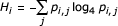where pi,j is the estimated probability of nucleotide j at position i.

The estimated mutual information (MI)  between two positions, i and i', is computed as: MIi,i' = H i + Hi' - Hi,i', where H i is the estimated entropy computed from the frequency of the four nucleotides at position i and Hi,i' is the entropy computed using the frequency of the 16 pairs of nucleotides at the two positions.

Nucleotide and nucleotide pair probabilities are themselves estimated using the Bayesian posterior mean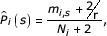where mi,s is the frequency of nucleotide or nucleotide pair s in position(s) i of the alignment, N i is the total number of sequences in the alignment at position(s) i, and r is the number of distinct classes in the probability distribution (for single nucleotides r = 4, for nucleotide pairs, r = 16, and so on).

Under the null hypothesis of no correlation between nucleotide positions in RSS, positive MIi,i' values may still be observed. To test if the MIi,i' values differ significantly from 0, we randomized the order of the sequences in position i' and recomputed MIi,i'. By randomizing the order of the sequences in one position but not the other, we preserve both marginal distributions but disrupt any correlations. The proportion of 300 permutations giving MIi,i' values higher than that observed in the data is our estimate for the p value under the null hypothesis.

### Statistical models of RSS structure

Single models for 12-RSS and 23-RSS define the set of probability distributions that contain the most information about RSS structure. We developed the models by stepwise model enlargement and selection. We begin with the smallest models, order zero Markov models estimated under the assumption of pairwise site independence. These models take as the probability of observing a sequence S, the product of the individual marginal probabilities over the RSS positions, P(S) = Π i P i (s) where s is the nucleotide observed in sequence S at position i. The marginal probability distribution for a position defines the probability of observing each of the four nucleotides at that position and is estimated from the RSS dataset using the Bayesian estimator for P i (s) described above. Each potential model enlargement is accomplished by replacing the product of an independent marginal probability and k-variate joint probability by a corresponding k + 1-variate joint probability.

For example, the first step of model enlargement for RSS of length N compares all possible combinations of one joint probability distribution for two positions and marginal probability distributions for the remaining N-2. positions: for every pair of positions i and i', there is the model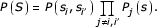Under each of these models we compute scores by taking the natural logarithm of P(S) (ln P(S)) for each RSS in the dataset using leave-one-out cross-validation . Briefly, for each model, we exclude one RSS from the dataset, estimate the probability distributions used in the model from the remaining RSS, and compute ln P(S) for the excluded RSS. We perform this computation for each RSS and take the average ln P(S) over all RSS. We then select the combination of probability distributions giving the largest average ln P(S).

The second step of model enlargement expands the model selected in step one by comparing all models within two classes: those based on two joint probability distributions, the one formed in step one and one for an additional pair of positions, and marginal probability distributions for the remaining N - 4 positions, and those based on one joint probability distribution for a group of three positions, the pair joint selected in step one expanded to include one additional position, and marginal probability distributions for the remaining N - 3 positions. We again compute ln P(S) under each model for each RSS using leave-one-out cross-validation and select the model giving the largest average ln P(S).

We continued the step-wise model enlargement and selection, iteratively expanding the models until the mean ln P(S) ceased improving. The final model is not necessarily optimal, however; we perform the maximization in a step-wise manner and so, as in any stepwise statistical procedure, are not guaranteed to achieve the global maximum.

Once the final set of probability distributions has been selected (the mean ln P(S) no longer improves), the parameters of each probability distribution are estimated on the complete set of RSS. ln P(S) for an RSS is a value between - ∞ and 0. If RSS were strictly conserved, the consensus RSS would have ln P(S) = 0. We define the ln P(S) of a sequence computed from the final model as its RSS information content (RIC). The final models take the form:

RIC12 = ln [P1P2P3,15,25P4,5P6,28P7,8,19P9,26P10,12P11,27P13,14,23P16,17,18P20,21,22P24]

and

RIC23 = ln [P1P2P3P4,14P5,39P6P7,24,25P8,9,21P10,16P11,12P13,22P15,23P17,18P19,27,30,31,32,33,37P20,26P28,29P34,38P35,36].

The CA dinucleotide at positions 1 and 2 of the heptamer is required for rearrangement [46,52,68]. Therefore, the models assign a probability of 0 to any RSS not beginning with CA.

RSS in the dataset receive higher RIC values (and WM0 and WM1 values, see below) during genome searches than during model development, because the sequence for which RIC is calculated is excluded from the dataset during model development, a property of leave-one-out cross-validation . In contrast, nucleotide frequencies used in the searches are estimated on the complete set of RSS. The difference between the two RIC values is greatest for rare sequences and, in general, the differences are larger for 23-RSS. The difference between the two scores is < 1 for 78% of 12-RSS and for 39% of 23-RSS.

### Markov models

We also modeled RSS with weight matrices based on an order zero or order one Markov model (reviewed in ). The order zero Markov model assumes that all RSS positions are independent, so the probability of observing a sequence S that is N-bp long is: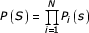where P i (s) is the probability of observing nucleotide s at RSS position i. P i (s) is estimated as described above from the set of physiologic 12- or 23-RSS. The score for sequence S is then the natural logarithm of the probability P(S),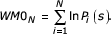The order one Markov model assumes that all adjacent positions are correlated, so the probability of observing sequence S is based on conditional probabilities: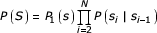where P(s i |si-1) is the probability of observing nucleotide s i in position i given that nucleotide si-1 occupies position i - 1. From Bayes rule,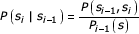where P(si-1,s i ,) is the joint probability distribution for the dinucleotide si-1s i occurring at the pair of positions i - 1 and i. Again, the probability distributions are estimated from the physiologic 12- or 23-RSS, and we take the natural logarithm of the probability P(S) as the score for sequence S: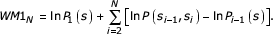## References

1. 1.

Mitchison A: Partitioning of genetic variation between regulatory and coding gene segments: the predominance of software variation in genes encoding introvert proteins. Immunogenetics. 1997, 46: 46-52. 10.1007/s002510050241.

2. 2.

Purugganan MD: The molecular population genetics of regulatory genes. Mol Ecol. 2000, 9: 1451-1461. 10.1046/j.1365-294x.2000.01016.x.

3. 3.

Kolchanov NA, Ignatieva EV, Ananko EA, Podkolodnaya OA, Stepanenko IL, Merkulova TI, Pozdnyakov MA, Podkolodny NL, Naumochkin AN, Romashchenko AG: Transcription Regulatory Regions Database (TRRD): its status in 2002. Nucleic Acids Res. 2002, 30: 312-317. 10.1093/nar/30.1.312.

4. 4.

Zhu J, Zhang MQ: SCPD: a promoter database of the yeast Saccharomyces cerevisiae. Bioinformatics. 1999, 15: 607-611. 10.1093/bioinformatics/15.7.607.

5. 5.

Vanet A, Marsan L, Sagot MF: Promoter sequences and algorithmical methods for identifying them. Res Microbiol. 1999, 150: 779-799. 10.1016/S0923-2508(99)00115-1.

6. 6.

Perier RC, Praz V, Junier T, Bonnard C, Bucher P: The eukaryotic promoter database (EPD). Nucleic Acids Res. 2000, 28: 302-303. 10.1093/nar/28.1.302.

7. 7.

Wingender E, Chen X, Hehl R, Karas H, Liebich I, Matys V, Meinhardt T, Pruss M, Reuter I, Schacherer F: TRANSFAC: an integrated system for gene expression regulation. Nucleic Acids Res. 2000, 28: 316-319. 10.1093/nar/28.1.316.

8. 8.

Bucher P: Weight matrix descriptions of four eukaryotic RNA polymerase II promoter elements derived from 502 unrelated promoter sequences. J Mol Biol. 1990, 212: 563-578.

9. 9.

Walker M, Pavlovic V, Kasif S: A comparative genomic method for computational identification of prokaryotic translation initiation sites. Nucleic Acids Res. 2002, 30: 3181-3191. 10.1093/nar/gkf423.

10. 10.

Day WH, McMorris FR: Critical comparison of consensus methods for molecular sequences. Nucleic Acids Res. 1992, 20: 1093-1099.

11. 11.

Stormo GD, Schneider TD, Gold L, Ehrenfeucht A: Use of the 'Perceptron' algorithm to distinguish translational initiation sites in E. coli. Nucleic Acids Res. 1982, 10: 2997-3011.

12. 12.

Harr R, Haggstrom M, Gustafsson P: Search algorithm for pattern match analysis of nucleic acid sequences. Nucleic Acids Res. 1983, 11: 2943-2957.

13. 13.

Stormo GD: Consensus patterns in DNA. Methods Enzymol. 1990, 183: 211-221.

14. 14.

Stormo GD: DNA binding sites: representation and discovery. Bioinformatics. 2000, 16: 16-23. 10.1093/bioinformatics/16.1.16.

15. 15.

Stormo GD, Schneider TD, Gold L: Quantitative analysis of the relationship between nucleotide sequence and functional activity. Nucleic Acids Res. 1986, 14: 6661-6679.

16. 16.

Berg OG, von Hippel PH: Selection of DNA binding sites by regulatory proteins. Statistical-mechanical theory and application to operators and promoters. J Mol Biol. 1987, 193: 723-750.

17. 17.

Fickett JW: Quantitative discrimination of MEF2 sites. Mol Cell Biol. 1996, 16: 437-441.

18. 18.

Lustig B, Jernigan RL: Consistencies of individual DNA base-amino acid interactions in structures and sequences. Nucleic Acids Res. 1995, 23: 4707-4711.

19. 19.

Roulet E, Fisch I, Junier T, Bucher P, Mermod N: Evaluation of computer tools for the prediction of transcription factor binding sites on genomic DNA. In Silico Biol. 1998, 1: 21-28.

20. 20.

Roulet E, Bucher P, Schneider R, Wingender E, Dusserre Y, Werner T, Mermod N: Experimental analysis and computer prediction of CTF/NFI transcription factor DNA binding sites. J Mol Biol. 2000, 297: 833-848. 10.1006/jmbi.2000.3614.

21. 21.

Stormo GD, Strobl S, Yoshioka M, Lee JS: Specificity of the Mnt protein. Independent effects of mutations at different positions in the operator. J Mol Biol. 1993, 229: 821-826. 10.1006/jmbi.1993.1088.

22. 22.

Takeda Y, Sarai A, Rivera VM: Analysis of the sequence-specific interactions between Cro repressor and operator DNA by systematic base substitution experiments. Proc Natl Acad Sci USA. 1989, 86: 439-443.

23. 23.

Tronche F, Ringeisen F, Blumenfeld M, Yaniv M, Pontoglio M: Analysis of the distribution of binding sites for a tissue-specific transcription factor in the vertebrate genome. J Mol Biol. 1997, 266: 231-245. 10.1006/jmbi.1996.0760.

24. 24.

Bulyk ML, Johnson PL, Church GM: Nucleotides of transcription factor binding sites exert interdependent effects on the binding affinities of transcription factors. Nucleic Acids Res. 2002, 30: 1255-1261. 10.1093/nar/30.5.1255.

25. 25.

Salzberg SL: A method for identifying splice sites and translational start sites in eukaryotic mRNA. Comput Appl Biosci. 1997, 13: 365-376.

26. 26.

Zhang MQ, Marr TG: A weight array method for splicing signal analysis. Comput Appl Biosci. 1993, 9: 499-509.

27. 27.

Burge CB: Modeling dependencies in pre-mRNA splicing signals. In Computational Methods in Molecular Biology. Edited by: Salzberg SL, Searls DB Kasif S. 1998, New York: Elsevier, 371-

28. 28.

Ponomarenko MP, Ponomarenko JV, Frolov AS, Podkolodnaya OA, Vorobyev DG, Kolchanov NA, Overton GC: Oligonucleotide frequency matrices addressed to recognizing functional DNA sites. Bioinformatics. 1999, 15: 631-643. 10.1093/bioinformatics/15.7.631.

29. 29.

Zazopoulos E, Lalli E, Stocco DM, Sassone-Corsi P: DNA binding and transcriptional repression by DAX-1 blocks steroidogenesis. Nature. 1997, 390: 311-315. 10.1038/36899.

30. 30.

Bianchi ME, Beltrame M, Paonessa G: Specific recognition of cruciform DNA by nuclear protein HMG1. Science. 1989, 243: 1056-1059.

31. 31.

Robbe K, Bonnefoy E: Non-B-DNA structures on the interferon-beta promoter?. Biochimie. 1998, 80: 665-671. 10.1016/S0300-9084(99)80020-0.

32. 32.

Wadkins RM: Targeting DNA secondary structures. Curr Med Chem. 2000, 7: 1-15.

33. 33.

Sadofsky MJ: The RAG proteins in V(D)J recombination: more than just a nuclease. Nucleic Acids Res. 2001, 29: 1399-1409. 10.1093/nar/29.7.1399.

34. 34.

Burge C, Karlin S: Prediction of complete gene structures in human genomic DNA. J Mol Biol. 1997, 268: 78-94. 10.1006/jmbi.1997.0951.

35. 35.

Cai D, Delcher A, Kao B, Kasif S: Modeling splice sites with Bayes networks. Bioinformatics. 2000, 16: 152-158. 10.1093/bioinformatics/16.2.152.

36. 36.

Zinkernagel RM, Hengartner H: Regulation of the immune response by antigen. Science. 2001, 293: 251-253. 10.1126/science.1063005.

37. 37.

Janeway C, Travers P, Walport M, Shlomchik M: Immunobiology: The Immune System in Health and Disease. 2001, New York: Garland

38. 38.

Radic MZ, Weigert M: Origins of anti-DNA antibodies and their implications for B-cell tolerance. Ann NY Acad Sci. 1995, 764: 384-396.

39. 39.

Sprent J, Kishimoto H: T cell tolerance and the thymus. Ann NY Acad Sci. 1998, 841: 236-245.

40. 40.

Davila M, Foster S, Kelsoe G, Yang K: A role for secondary V(D)J recombination in oncogenic chromosomal translocations?. Adv Cancer Res. 2001, 81: 61-92.

41. 41.

Fugmann SD, Lee AI, Shockett PE, Villey IJ, Schatz DG: The RAG proteins and V(D)J recombination: complexes, ends, and transposition. Annu Rev Immunol. 2000, 18: 495-527. 10.1146/annurev.immunol.18.1.495.

42. 42.

Difilippantonio MJ, McMahan CJ, Eastman QM, Spanopoulou E, Schatz DG: RAG1 mediates signal sequence recognition and recruitment of RAG2 in V(D)J recombination. Cell. 1996, 87: 253-262.

43. 43.

Sakano H, Huppi K, Heinrich G, Tonegawa S: Sequences at the somatic recombination sites of immunoglobulin light-chain genes. Nature. 1979, 280: 288-294.

44. 44.

Tonegawa S: Somatic generation of antibody diversity. Nature. 1983, 302: 575-581.

45. 45.

Lewis SM: The mechanism of V(D)J joining: lessons from molecular, immunological, and comparative analyses. Adv Immunol. 1994, 56: 27-150.

46. 46.

Hesse JE, Lieber MR, Mizuuchi K, Gellert M: V(D)J recombination: a functional definition of the joining signals. Genes Dev. 1989, 3: 1053-1061.

47. 47.

Feeney AJ, Tang A, Ogwaro KM: B-cell repertoire formation: role of the recombination signal sequence in non-random V segment utilization. Immunol Rev. 2000, 175: 59-69.

48. 48.

Livak F, Petrie HT: Somatic generation of antigen-receptor diversity: a reprise. Trends Immunol. 2001, 22: 608-612. 10.1016/S1471-4906(01)02054-3.

49. 49.

Lewis SM, Agard E, Suh S, Czyzyk L: Cryptic signals and the fidelity of V(D)J joining. Mol Cell Biol. 1997, 17: 3125-3136.

50. 50.

Marculescu R, Le T, Simon P, Jaeger U, Nadel B: V(D)J-mediated translocations in lymphoid neoplasms: a functional assessment of genomic instability by cryptic sites. J Exp Med. 2002, 195: 85-98. 10.1084/jem.20011578.

51. 51.

Kullback S: Information Theory and Statistics. 1959, New York: Wiley

52. 52.

Akamatsu Y, Tsurushita N, Nagawa F, Matsuoka M, Okazaki K, Imai M, Sakano H: Essential residues in V(D)J recombination signals. J Immunol. 1994, 153: 4520-4529.

53. 53.

Swanson PC, Desiderio S: V(D)J recombination signal recognition: distinct, overlapping DNA- protein contacts in complexes containing RAG1 with and without RAG2. Immunity. 1998, 9: 115-125.

54. 54.

Miller J, Selsing E, Storb U: Structural alterations in J regions of mouse immunoglobulin lambda genes are associated with differential gene expression. Nature. 1982, 295: 428-430.

55. 55.

Chen F, Rowen L, Hood L, Rothenberg EV: Differential transcriptional regulation of individual TCR V segments before gene rearrangement. J Immunol. 2001, 166: 1771-1780.

56. 56.

Chou HS, Anderson SJ, Louie MC, Godambe SA, Pozzi MR, Behlke MA, Huppi K, Loh DY: Tandem linkage and unusual RNA splicing of the T-cell receptor beta-chain variable-region genes. Proc Natl Acad Sci USA. 1987, 84: 1992-1996.

57. 57.

Lefranc MP: IMGT, the international ImMunoGeneTics database. Nucleic Acids Res. 2001, 29: 207-209. 10.1093/nar/29.1.207.

58. 58.

Kouskoff V, Nemazee D: Role of receptor editing and revision in shaping the B and T lymphocyte repertoire. Life Sci. 2001, 69: 1105-1113. 10.1016/S0024-3205(01)01219-X.

59. 59.

Kleinfield R, Hardy RR, Tarlinton D, Dangl J, Herzenberg LA, Weigert M: Recombination between an expressed immunoglobulin heavy-chain gene and a germline variable gene segment in a Ly 1+ B-cell lymphoma. Nature. 1986, 322: 843-846.

60. 60.

Usuda S, Takemori T, Matsuoka M, Shirasawa T, Yoshida K, Mori A, Ishizaka K, Sakano H: Immunoglobulin V gene replacement is caused by the intramolecular DNA deletion mechanism. EMBO J. 1992, 11: 611-618.

61. 61.

Chen C, Nagy Z, Prak EL, Weigert M: Immunoglobulin heavy chain gene replacement: a mechanism of receptor editing. Immunity. 1995, 3: 747-755.

62. 62.

Downloads for statistical models of recombination signal sequences that identify cryptic signals and predict recombination efficiencies. [http://www.duke.edu/~lgcowell]

63. 63.

Lefranc MP, Giudicelli V, Ginestoux C, Bodmer J, Muller W, Bontrop R, Lemaitre M, Malik A, Barbie V, Chaume D: IMGT, the international ImMunoGeneTics database. Nucleic Acids Res. 1999, 27: 209-212. 10.1093/nar/27.1.209.

64. 64.

Ruiz M, Giudicelli V, Ginestoux C, Stoehr P, Robinson J, Bodmer J, Marsh SG, Bontrop R, Lemaitre M, Lefranc G, et al: IMGT, the international ImMunoGeneTics database. Nucleic Acids Res. 2000, 28: 219-221. 10.1093/nar/28.1.219.

65. 65.

Shannon CE, Weaver W: The Mathematical Theory of Communication. 1949, Urbana, IL: University of Illinois Press

66. 66.

Jaynes ET: Probability Theory: The Logic Of Science. Edited by: Bretthorst GL. Cambridge: Cambridge University Press

67. 67.

Stone M: Cross-validatory choice and assessment of statistical predictions (with discussion). J Roy Stat Soc B. 1974, 36: 111-147.

68. 68.

Ramsden DA, Baetz K, Wu GE: Conservation of sequence in recombination signal sequence spacers. Nucleic Acids Res. 1994, 22: 1785-1796.

69. 69.

RasMol. [http://www.umass.edu/microbio/rasmol/]

## Acknowledgements

This work was supported in part by grants AI24335 and AI49326 (G.K.). L.G.C. received a Bioinformatics and Genome Technology Postdoctoral Fellowship from Duke University.

## Author information

Correspondence to Garnett Kelsoe.

## Rights and permissions

Reprints and Permissions

Cowell, L.G., Davila, M., Kepler, T.B. et al. Identification and utilization of arbitrary correlations in models of recombination signal sequences. Genome Biol 3, research0072.1 (2002) doi:10.1186/gb-2002-3-12-research0072

• #### DOI

https://doi.org/10.1186/gb-2002-3-12-research0072

### Keywords

• Gene Segment
• Model Selection Procedure
• Recombination Efficiency
• Recombination Signal Sequence
• Marginal Probability Distribution